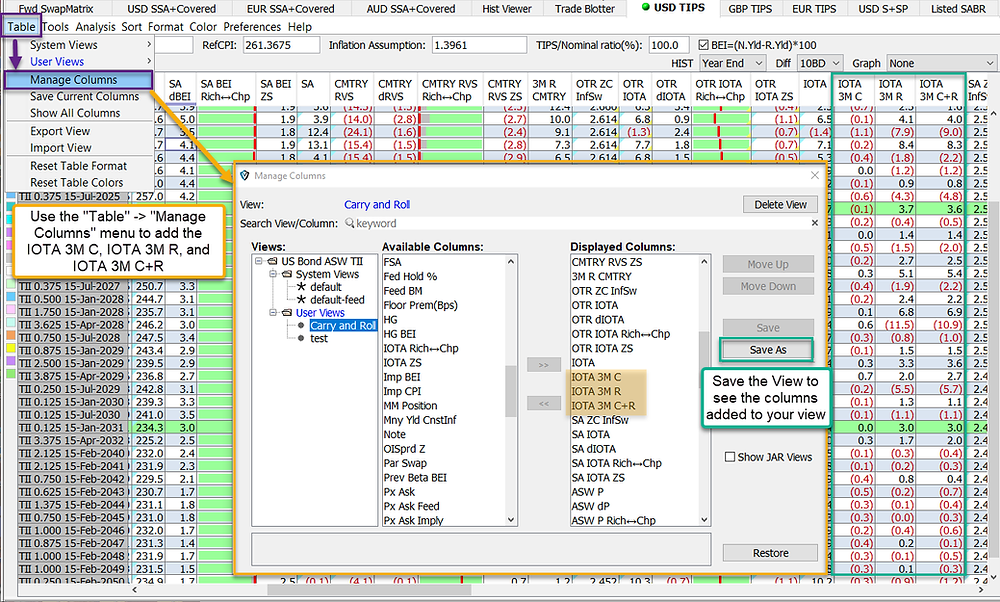# Tips Enhancements | Weekly Release 3/26/21

## Enhancement #1

Added Implied Breakeven, Implied CPI, TIPS Implied Repo and Treasury Implied Repo

Implied BEI and Implied CPI Calculation

Assume we have an inflation bond (TII), and a benchmark treasury bond (T).

The “Implied BEI” and “Implied CPI” are calculated based on the future values being equal for both the inflation bond and the corresponding treasury bond.

1. Assuming spending same amount of money on both bonds. That is, if we hold 100mm on TII, the notional of T is calculate as follows:2. Based on the cashflows of T, we can calculate the future value at TII maturity date using the treasury repo (RiskVal calls this the “Trsy Imp Repo”).

3. Assume a flat inflation X after latest CPI print + seasonality factors, we can derive TII nominal cashflows. Then we can calculate the future value at TII maturity date using inflation bond repo (RiskVal calls this the “TIPS Imp Repo”).

4. X can be solved such that future value of T and future value of TII are same, and we call it “Implied BEI”.

5. Based on X, we can calculate CPI at TII maturity date, and we call it “Implied CPITraders have the option to override either the Treasury Imp Repo or the TIPS Imp Repo to recalculate the Implied BEI and the Implied CPI

## Enhancement #2

Added 3M IOTA carry, 3M IOTA roll, and 3M IOTA carry and rollTraders can use the Table -> Manage columns menu to add the IOTA carry, IOTA roll, and IOTA Carry and Roll.